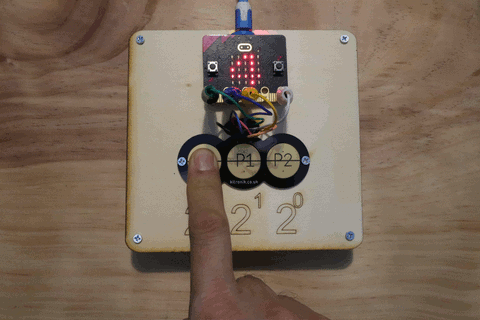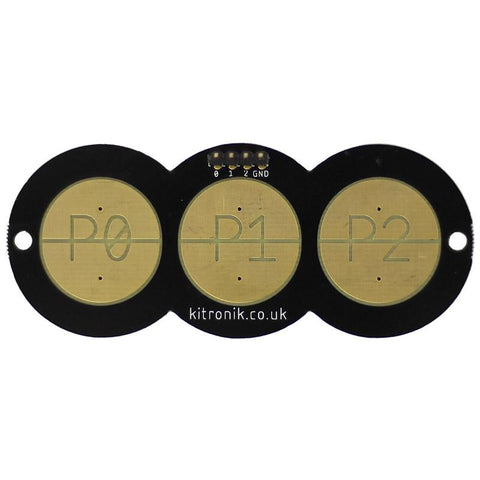# Project Tutorial: Make a number base Converter

BBC microbit BBC microbit v2 button kitronik mathematics microbit tutorials programming python#### In this project, you will learn:

• What number bases are
• How to connect and program buttons on the BBC micro:bit (works on V1 and V2)
• How to program the micro:bit in Python, to convert between different number bases

### Step 1: Number Bases

Number bases refer to the place value of each column in a number. We commonly use base10 during everyday life, which means that the place value of each column in base10 is a power of 10.

The top row of the table below, shows the place value for each column of the number 2,313 in base10. Each column value is multiplied by the number below it. So, the 2 in the 103 column is 10 x 10 x 10 x 2, because 103 = 10 x 10 x 10.

103 102 101 100
2 3 1 3

Because we are using base10, the highest number in a column is 9.

In the table below, we will represent the value of 25 in base10, as base2.

24 23 22 21 20
1 1 0 0 1

For base2, the highest number in a column can only be 1.

So if we work out the place value, 24 is 2 x 2 x 2 x 2 = 16 in base10. For the 23 column, we have 2 x 2 x 2 = 8, and for the 20 we have 1. Adding these together we have 16 + 8 + 1 = 25 in base10.

### Step 2: Connecting Switches to the micro:bit

The micro:bit has a ground pin (GND) and when we connect a wire between pins 0, 1, 2 to GND, the Python function, `is_touched()` will return a True value.

A code example below will display a happy face on the micro:bit when pin0 is connected to GND, and a sad face if it isn’t.

``````from microbit import *

while True: # keep looping forever
if pin0.is_touched():
display.show(Image.HAPPY)
else:
``````

You can test this by using flashing this code to your micro:bit and using a small wire to connect pin0 to the GND pin.

### Step 3: Using Switches to Represent Binary Numbers

Because a switch and be either on of off, it can be used to represent a single binary digit with 1 (on) or 0 (off).

Because the Kitronik Resistive Touch Keypad has 3 switches (pads that short together when touched), its perfect to represent a 3-digit binary (base2) number. We will connect each of the Resistive Touch Keypad pins to pins 0, 1, and 2 of the micro:bit and the keypad GND pin to the corresponding GND pin on the micro:bit.Note: capacitive touch, which doesn't require a connection to GND, is already implemented in the v2 hardware. At the time of writing, this was not yet implemented in the stable version of micro:bit Python. You can try this out in the development version of micro:bit Python here. This will just require an extra command to change the mode from resistive to capacitive i.e. `pin.set_touch_mode(mode)`.

To start off writing our Python script, we can use variables to set the place value powers.

``````from microbit import *

column_zero_power = 0
column_one_power = 1
column_two_power = 2
``````

Now, using the same code from before, repeated for each pin, we'll calculate each binary column value, and add it to our final result, `my_integer`.

``````while True:
my_integer, zero_binary, one_binary, two_binary = 0, 0, 0, 0 # reset everything before touch

if pin2.is_touched():
zero_binary = 1 # binary column 2 ^ 0
my_integer += 2 ** column_zero_power # add to decimal conversion

if pin1.is_touched():
one_binary = 1 # binary column 2 ^ 1
my_integer += 2 ** column_one_power # add to decimal conversion

if pin0.is_touched():
two_binary = 1 # binary column 2 ^ 2
my_integer += 2 ** column_two_power # add to decimal conversion
``````

We still need to display our result. This following code will display a message on the micro:bit as well as a message on the REPL debug console (accessible if you are using mu editor or Thonny). The following code will do this. There’s a `sleep()` command at the end to give the micro:bit some time to do catch up.

``````    print("Binary (base2): {}{}{} Decimal (base10): {}".format(two_binary, one_binary, zero_binary, my_integer))
display.show(my_integer) # also write it to the onboard LED matrix
sleep(100)
``````

Flash your program onto your micro:bit and press different combinations of the pads. You should see the equivalnt base10 number displayed on the micro:bit LED matrix.

### The Complete Program

The complete program is shown below:

``````from microbit import *

column_zero_power = 0
column_one_power = 1
column_two_power = 2

while True:
my_integer, zero_binary, one_binary, two_binary = 0, 0, 0, 0 # reset everything before touch

if pin2.is_touched():
zero_binary = 1 # binary column 2 ^ 0
my_integer += 2 ** column_zero_power # add to decimal conversion

if pin1.is_touched():
one_binary = 1 # binary column 2 ^ 1
my_integer += 2 ** column_one_power # add to decimal conversion

if pin0.is_touched():
two_binary = 1 # binary column 2 ^ 2
my_integer += 2 ** column_two_power # add to decimal conversion

print("Binary (base2): {}{}{} Decimal (base10): {}".format(two_binary, one_binary, zero_binary, my_integer))
display.show(my_integer) # also write it to the onboard LED matrix
sleep(100)``````

Each touched pad is read as a `1` with the remaining pads read as `0`. You can see how the micro:bit displays the base10 version of the binary numbers below.### Challenge

See if you can convert from base2 to base8 instead of base10.
Hint: You can use the `oct()` function to convert from base10 to base8 (octal).